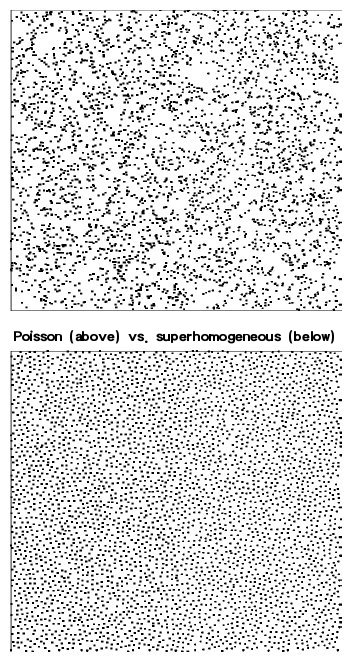# Matter density fields in the early universe

The most prominent feature of the initial conditions of the matter spatial distribution in the early universe, in standard theoretical models, derived from inflationary mechanisms, is that matter density field presents on large scale super-homogeneous features. This means the following. If one considers the paradigm of uniform distributions, the Poisson process where particles are placed completely randomly in space, the mass fluctuations in a sphere of radius R growths as the volume of the sphere.

A super-homogeneous distribution is a system where the average density is well defined and spatially constant (i.e., it is spatially homogeneous and uniform on large scales) and where mass fluctuations in a sphere grow slower than in the Poisson case, like the surface of the spherical volume of radius R. In other words a super-homogeneous stochastic mass distribution is more uniform than a random matter distribution and show a certain “level of order” at large scales.A well known system in statistical physics systems of this kind is the one component plasma (OCP) which is characterized by a dynamics which at thermal equilibrium, at sufficiently high temperatures, gives rise to such configurations. The OCP is simply a system of charged point particles interacting through a repulsive 1/r potential, in a uniform background which gives overall charge neutrality. Simple modifications of the OCP can produce equilibrium correlations of the kind assumed in the cosmological context.

Since the dynamical evolution of cosmological n-body simulations, under the effect of pure gravitation, strongly depends on the fine tuning of suitable initial conditions, the mathematical study of super-homogeneous particle distribution plays an important role in comparing theoretical results from fluid-like gravitational theory and the evolution of discrete n-body simulations. Moreover it is important to investigate the subject related to the possible existence of a maximal degree of super-homogeneity (i.e., stochastic order) beyond which the features of the gravitational structure formation from initial fluctuations changes dramatically to a sort of local dynamics.
References

 A. Gabrielli, F. Sylos Labini, M. Joyce, and L. Pietronero, “STATISTICAL PHYSICS FOR COSMIC STRUCTURES”, Springer Verlag Inc. (New York-Berlin, 2004).

 A. Gabrielli, B. Jancovici, M. Joyce, J. L. Lebowitz, L. Pietronero, and F. Sylos Labini, “Generation of primordial cosmological perturbations from statistical mechanical models”, Phys. Rev. D, 67, 043506 (2003).

 R. Durrer, A. Gabrielli, M. Joyce, F. Sylos Labini, “Bias and the power spectrum beyond the turn-over”, Astrphysical Jour. Lett., 585, L1-L4 (2003).

 A. Gabrielli, M. Joyce, B. Marcos, and P. Viot, “Causality constraints of fluctuations in cosmology: a study with exactly solvable one dimensional models”, Europhys. Lett., 66, 1 (2004).

 F. Sylos Labini, A. Gabrielli, “Complexity in cosmic structures”, Physica A, 338/1-2, 44 (2004).

 A. Gabrielli and S. Torquato, “Voronoi and Voids Statistics for Super-homogeneous Point Processes”, Phys. Rev. E, 70, 041105 (2004)

 A. Gabrielli, “Point processes and stochastic displacement fields”, Phys. Rev. E., 70, 066131 (2004).

 A. Gabrielli and M. Joyce, “Two-point correlation properties of stochastic splitting processes ”, Phys. Rev. E, 77, 031139 (2008).

 A. Gabrielli, M. Joyce, and S. Torquato, “Tilings of space and superhomogeneous point processes”, Phys. Rev. E, 77, 031125 (2008).

Tags: